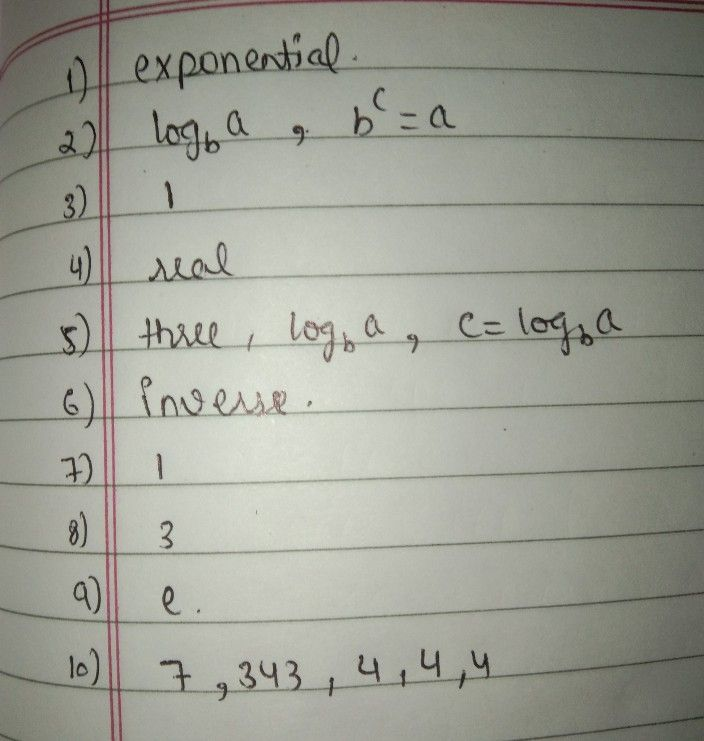Symbol
Problem$omPlct$ the following statements by writing the correct word or words $an4$ formulas. 1. Logarithm is the inverse of 2. $rhc$ logarithm of a with base b is denoted by and is defined as $c=log _{b}ai$ and only if 3. In logarithmic form logb a the value of b cannot be $4.$ $Tha$ value of log a can be $5$ Let a, b, and c be real numbers such that $b≠1$ The logarithm $ofa$ with base b is denoted by and is defined as if and only $ifa=b^{c}$ 6. Logarithmic functions and exponential functions are 7. In logarithmic form log, a, b cannot be 8. $Th$ base in the given logarithmic expression $log _{3}5$ is 9. If the base is not written in the logarithmic expression, then it is understood. be $10.$ From the given $log _{7}343$ it is the same as asking "What will be the exponent of to $gc$ ? Since $7-=343T$ 343Therefore, $log _{7}343$ = $log _{7}343=$ then,